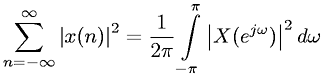Equations > Signal Processing > Fourier Transform Properties > Discrete-Time Fourier transform - Parseval's Theorem

### Discrete-Time Fourier transform - Parseval's TheoremLatex Code:

MathML Code:

 $\sum _{n=-\infty }^{\infty }{|x\left(n\right)|}^{2}=\frac12\pi \underset{-\pi }{\overset{\pi }{\int }}{|X\left({e}^{j\omega }\right)|}^{2}d\omega$

MathType 5.0: